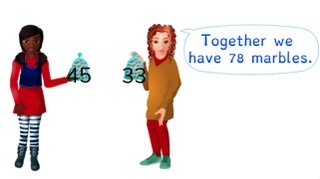Mental math + - to 100

# Mental math + - to 100

Practice mental math of addition and subtraction problems to 100.

No account needed.8,000 schools use Gynzy92,000 teachers use Gynzy1,600,000 students use Gynzy

## General

Students learn to use mental math to solve addition and subtraction problems to 100.

## Standards

CCSS.Math.Content.2.NBT.B.5

## Learning objective

Students will be able to solve addition and subtraction problems to 100 in their heads.

## Introduction

Have students find the animals in the background with the spotlight and solve the math problems that they have.

## Instruction

Tell students that when solving a math problem using mental math, that means solving it without writing it down on a piece of scratch paper. Start by looking carefully at the numbers. In the example you see that the 6 and 3 are in the tens place and the 8 and 0 in the ones places. Add the numbers. Show another math problem, and explain that there are different ways in which to solve the math problem. Ask students to discuss possible strategies for solving the problems in pairs. Then show a selection of problems for students to solve individually or in pairs in their heads.
Subtraction problems can be solved in the same way as addition problems. Start by looking carefully at the number. Have students take the first number and then split the second number and take away the tens and the ones from the first number. Or you can also choose to calculate how much you need to add to a number to reach the nearest tens (go from 68 to 70). Ask students to solve a few problems, making sure to discuss possible strategies. Then explain how to solve a story problem. Again, ask the students to practice solving the problems in their heads.

Check that students are able to solve addition and subtraction problems to 100 in their heads by asking the following question"
- Why is it useful to be able to use mental math on addition and subtraction problems to 100?

## Quiz

Students are given mental math problems involving addition or subtraction. They are then asked to also solve story problems with addition or subtraction.

## Closing

Discuss with students that it is important to be able to solve math problems in your head, so you can determine totals and differences without using a calculator or piece of scratch paper. To close ask students to come up with three addition or subtraction problems and to exchange them with a partner- solving each other's problems using mental math.

## Teaching tips

Students who have difficulty solving addition and subtraction problems in their head can start by solving them on a sheet of scratch paper. Have them practice a variety of strategies to solve the problems to make them more comfortable with the process. Then show them a problem and have them discuss how they would solve the problem. See if they can do the steps of solving the problem without writing them down. Start with easier problems and progress to more complex problems.

### The online teaching platform for interactive whiteboards and displays in schools

• Save time building lessons

• Manage the classroom more efficiently

• Increase student engagement